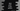# Java program to print X star pattern## Java program to print X star pattern

Learn to print the X pattern with stars in Java. We will learn the algorithm and the Java program to print the X pattern in this blog post. This is a beginner-level Java programming question. We can use star or any other character to print this pattern. Let me first show you how the algorithm works.

### Algorithm to print X star pattern in Java:

The X pattern looks as below:

``````*           *
*       *
*   *
*
*   *
*       *
*           *``````

This pattern is of size 7. We are using blank spaces and stars to print this pattern. Let’s replace all blank spaces with #,

``````* # # # # # *
# * # # # * #
# # * # * # #
# # # * # # #
# # * # * # #
# * # # # * #
* # # # # # *``````

So,

• For the first line, we need to print stars at the first and last positions.
• For the second line, we need to print stars at the second and second last positions etc. For all other places, we have to print blank spaces.

We can use the following algorithm:

• Read the size of the pattern as input from the user.
• Run one loop from i = 0 to i = size - 1.
• Run another inner loop from j = 0 to j = size - 1.
• Inside the inner loop, print a star if the value of i is equal to j or j is equal to size - i - 1. Else, print a blank space.

### Example 1: Java program to print star X pattern with for loops:

Below is the complete program:

``````import java.util.Scanner;

public class Main {

public static void main(String[] args) {
Scanner scanner = new Scanner(System.in);

System.out.println("Enter the size of the pattern:");
int size = scanner.nextInt();

for (int i = 0; i < size; i++) {
for (int j = 0; j < size; j++) {
if (i == j || j == size - i - 1)
System.out.print("* ");
else
System.out.print("  ");
}
System.out.println();
}
}
}``````
• The size of the pattern is assigned to the size variable.
• The for loops are used to print the pattern as we discussed before.

It will print outputs as below:

``````Enter the size of the pattern:
6
*         *
*     *
* *
* *
*     *
*         *

Enter the size of the pattern:
7
*           *
*       *
*   *
*
*   *
*       *
*           * ``````

### Example 2: Java program to print star X pattern with while loops:

The following program uses while loops to print the X pattern with stars:

``````import java.util.Scanner;

public class Main {

public static void main(String[] args) {
Scanner scanner = new Scanner(System.in);
int i = 0, j;

System.out.println("Enter the size of the pattern:");
int size = scanner.nextInt();

while (i < size) {
j = 0;
while (j < size) {
if (i == j || j == size - i - 1)
System.out.print("* ");
else
System.out.print("  ");
j++;
}
System.out.println();
i++;
}
}
}``````

This program prints similar results. Make sure to initialize the variables used in the loops before the loop starts.

### Example 3: Java program to print the X pattern with any character:

We can always take the character as input from the user and print the pattern by using that character.

``````import java.util.Scanner;

public class Main {

public static void main(String[] args) {
Scanner scanner = new Scanner(System.in);

System.out.println("Enter the size of the pattern:");
int size = scanner.nextInt();

System.out.println("Enter the character to print the pattern:");
char c = scanner.next().charAt(0);

for (int i = 0; i < size; i++) {
for (int j = 0; j < size; j++) {
if (i == j || j == size - i - 1)
System.out.print(c + " ");
else
System.out.print("  ");
}
System.out.println();
}
}
}``````
• One new character variable, c is introduced in this program.
• It asks the user to enter the character to print the X pattern. It uses the scanner variable to read that value and assigns it to the variable c.
• Inside the loop, it prints the value of c in place of star.

It will print the X pattern with any character you provide:

``````Enter the size of the pattern:
5
Enter the character to print the pattern:
&
&       &
&   &
&
&   &
&       &

Enter the size of the pattern:
6
Enter the character to print the pattern:
#
#         #
#     #
# #
# #
#     #
#         # ``````